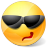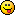# Math Is Fun Forum

Discussion about math, puzzles, games and fun.   Useful symbols: ÷ × ½ √ ∞ ≠ ≤ ≥ ≈ ⇒ ± ∈ Δ θ ∴ ∑ ∫  π  -¹ ² ³ °

You are not logged in.

## #1 2014-08-07 04:49:47

careless25
Real MemberRegistered: 2008-07-24
Posts: 560

### Correlation Coefficient

This was a question on my exam and I am not sure I did it correctly. Can someone show me the steps to derive it?

Given X1, X2, X3, X4 are random uncorrelated variables with variance of 1 and mean of 0. Find the correlation coefficient for (X1 + X2 and X2 + X3).

I got the answer as -1/2. is this correct?

Offline

## #2 2014-08-07 20:28:53

BobRegistered: 2010-06-20
Posts: 9,278

### Re: Correlation Coefficient

hi careless25,

I'm well out of my comfort zone with this one so what follows may be rubbish.

Intuitively, I feel it ought to be zero, as the variables are all uncorrelated.

So I thought I'd better check on the formulas:

http://www.math.uiuc.edu/~hildebr/461/variance.pdf

So we have V[X1] = V[X2] = V[X3] = V[X4] = 1

So V[X1+X2] = V[X3+X4] = 1 + 1 = 2

and V[X1+X2+X3+X4] = 4

So Cov[X1+X2.X3+X4] = 0.5(V[X1+X2+X3+X4] - V[X1+X2] - V[X3+X4]) = 0.5(4-2-2) = 0

P[X1+X2,X3+X4] = Cov[X1+X2.X3+X4] /(ROOT(V[X1+X2]*V[X3+X4])= 0/2 = 0

Bob

Children are not defined by school ...........The Fonz
You cannot teach a man anything;  you can only help him find it within himself..........Galileo Galilei
Sometimes I deliberately make mistakes, just to test you!  …………….BobOffline

## #3 2014-08-08 02:00:01

careless25
Real MemberRegistered: 2008-07-24
Posts: 560

### Re: Correlation Coefficient

Hi Bob Bundy,

I am asking for the Correlation coefficient of X1 + X2 and X2+X3. Not X1+X2 and X3+X4.

I agree with your answer if it was that.

Offline

## #4 2014-08-08 02:03:20

BobRegistered: 2010-06-20
Posts: 9,278

### Re: Correlation Coefficient

Oh sorry.  I'll recalculate.but it'll have to be a little later as I've got to log off now.

Bob

Children are not defined by school ...........The Fonz
You cannot teach a man anything;  you can only help him find it within himself..........Galileo Galilei
Sometimes I deliberately make mistakes, just to test you!  …………….BobOffline

## #5 2014-08-08 04:12:41

BobRegistered: 2010-06-20
Posts: 9,278

### Re: Correlation Coefficient

revised version 1:

V[X1] = V[X2] = V[X3] = V[X4] = 1

So V[X1+X2] = V[X2+X3] = 1 + 1 = 2

and V[X1+X2+X2+X3] = 4

So Cov[X1+X2.X2+X3] = 0.5(V[X1+X2+X2+X3] - V[X1+X2] - V[X2+X3]) = 0.5(4-2-2) = 0

P[X1+X2,X3+X3] = Cov[X1+X2.X2+X3] /(ROOT(V[X1+X2]*V[X2+X3])= 0/2 = 0

revised version 2:

and V[X1+X2+X2+X3] = V[X1+2X2+X3] = 1 + 4 + 1 = 6

So Cov[X1+X2.X2+X3] = 0.5(V[X1+X2+X2+X3] - V[X1+X2] - V[X2+X3]) = 0.5(6-2-2) = 1

P[X1+X2,X2+X3] = Cov[X1+X2.X2+X3] /(ROOT(V[X1+X2]*V[X2+X3])= 1/2

This feels better to me.  The X1+X2 and X2 + X3 variables have a 'bias' towards X2 so I'd expect them to correlate positively.

I'm going to try to construct a simulation to sort this out.  It may take a while ........................

Bob

Children are not defined by school ...........The Fonz
You cannot teach a man anything;  you can only help him find it within himself..........Galileo Galilei
Sometimes I deliberately make mistakes, just to test you!  …………….BobOffline

## #6 2014-08-08 05:41:21

BobRegistered: 2010-06-20
Posts: 9,278

### Re: Correlation Coefficient

Here's how I constructed the simulation:

(1)  I used MS Excel.

(2)  I used the function RAND() to generate numbers in the range 0 to 1.

(3) I used the function NORMSINV() to convert the number from step (2) into an x value from a Normal distribution, with mean=0, variance=1.

(4)  I copied the formulas into 200 rows.

(5)  To check that these values were as required I computed the mean and variance of the numbers.

(6) I repeated this for three more columns.

(7) In two more columns I computed X1+X2 and X2+X3.

(8) I made a scatter graph for these two columns and also computed the correlation coefficient.

This screen shot shows the last few rows, and the graph.Bob

Children are not defined by school ...........The Fonz
You cannot teach a man anything;  you can only help him find it within himself..........Galileo Galilei
Sometimes I deliberately make mistakes, just to test you!  …………….BobOffline

## #7 2014-08-08 05:43:33

careless25
Real MemberRegistered: 2008-07-24
Posts: 560

### Re: Correlation Coefficient

I must have messed up some of my signs somewhere.

Thanks Bob!

EDIT:

Actually how is V[X1+X2+X2+X3] = 6?? shouldn't it be 4 as V[X1 + 2*X2 + X3] = V[X1] + 2*V[X2] + V[X3] = 1+2+1?

Last edited by careless25 (2014-08-08 05:46:29)

Offline

## #8 2014-08-08 06:03:25

BobRegistered: 2010-06-20
Posts: 9,278

### Re: Correlation Coefficient

VAR[cX] = c^2.VAR[X]

It happens because you square the differences from the mean.

Bob

Children are not defined by school ...........The Fonz
You cannot teach a man anything;  you can only help him find it within himself..........Galileo Galilei
Sometimes I deliberately make mistakes, just to test you!  …………….BobOffline